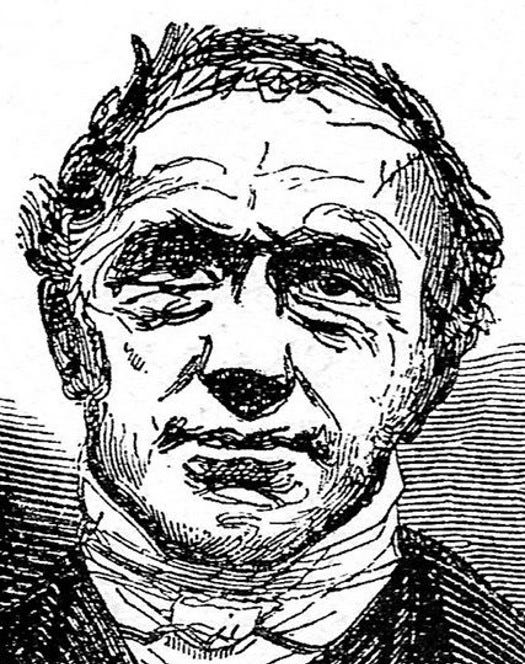# Mathematics of Choice — Adrien-Marie LegendrePortrait based on a sketch Legendre provided to Francois Arago in 1823, from Barral (1892).

Adrien-Marie Legendre introduced an approach for dealing with errors in measurements that remains one of the most important tools used by engineers and scientists. Legendre was part of a group of mathematicians, along with Laplace, Lagrange, and Monge, who laid the foundations for a more quantitative approach to science and engineering that took hold at the beginning of the 19th century. Legendre worked on predicting the trajectory of cannon balls, measuring the shape of the Earth, and astronomy. In all three areas, he faced a recurring problem — how to make the best choice among several alternatives based on data that are inherently contaminated by error.

Legendre was studying the problem of identifying the orbit of a comet when he seized on the approach known as the least squared error. Typically, astronomers have a small set of measurements of the position of a comet collected during the short time that it is near the sun and visible in the sky. From these data, astronomers want to identify the equation that describes the comet’s entire orbital path around the sun and predicts the next time the comet will be visible in the sky. The problem is that no equation fits the measured positions of the comet because each of the measurements is contaminated by the addition of errors. These errors arise from a variety of sources. Astronomers work hard to minimize errors in measurement, but inevitably they occur.

Legendre’s solution was to identify the orbital equation that best fits the data, taking error into account. For every possible orbit, Legendre determine the distance between the position calculated for the comet and the measured position recorded by the astronomers; these defined the set of errors. He then calculated the square of these distances, and summed them all up. The orbital equation that had the smallest total, the “least squares error,” was the one he chose.

The problem of choosing the best orbital equation has parallels in many other areas that involve the analysis of data, most of them quite different from determining the orbit of a comet. Therefore, others soon adopted Legendre’s method of least squares because it is easy to apply. And, it turns out to be the rationally-defensible solution to this general problem in a wide range of situations.

Adrien-Marie Legendre is one of the 72 scientist and engineers named on the Eiffel Tower.